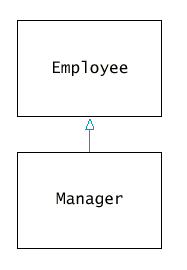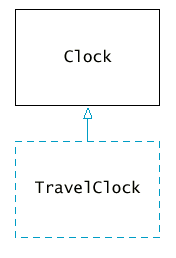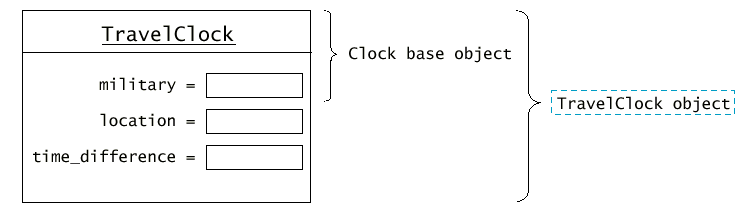Inheritance

## Chapter Goals

• To understand the concepts of inheritance and polymorphism
• To learn how inheritance is a tool for code reuse
• To learn how to call base-class constructors and member functions
• To understand the difference between static and dynamic binding
• To be able to implement dynamic binding with virtual functions

## Derived Classes

• Inheritance is a mechanism for enhancing existing working classes.
• If a new class needs to be implemented and a class representing a more general concept is already available, then the new class can inherit from the existing class.
• The existing, more general class is called the base class.
• The more specialized class that inherits from the base class is called the derived class.
• Example: We already have a class Employee, and a manager is a special case of an employee.
`class Manager : public Employee {public:   new member functionsprivate:   new data members};`

Syntax 11.1: Derived Class Definition

`class Derived_class_name : public Base_class_name{   features};`
 Example: `class Manager : public Employee { public: Manager(string name, double salary, string dept); string get_department() const; private: string department;};` Purpose: Define a class that inherits features from a base class.
• All member functions and data members of the base class are automatically inherited by the derived class.
`Manager m;m.set_salary(68000);`
• In the derived class definition you specify only new member functions and data members.
`class Manager : public Employee {public:   Manager(string name, double salary, string dept);   string get_department() const;private:   string department;};`
• The class diagram below shows the relationship between derived classes. The arrow with a hollow head indicates inheritance.• Example: Modeling a set of clocks that display the times in different cities.

## Derived Classes (clocks1.cpp)

• The Clock class provides a base class that can tell the current local time.
• You can set a clock to either "military" or "am/pm" format.
• We will create a derived class, TravelClock that gains two additional data fields: location and time_difference.• The time for a TravelClock is computed by taking the local time and adding the time difference. (For Time Zones see here.)
`TravelClock clock("London", -2);cout << "The time in " << clock.get_location() << " is "   << clock.get_hours() << ":" << clock.get_minutes();`
• A TravelClock differs from a Clock in three ways:
• Its objects store the location and time difference.
• The get_hours function of the TravelClock adds the time difference to the current time.
• The get_location function returns the actual location, not the string "Local".
• When the TravelClock class inherits from the Clock class, it needs only to spell out these three differences.
`class TravelClock : public Clock {public:   TravelClock(bool mil, string loc, double off);   int get_hours() const;   string get_location() const;private:   string location;   int time_difference;};`
• The data members of the base class are present in each derived class object, but they are not accessible by the member functions of the derived class because these fields are private data of the base class.

## Calling the Base-Class Constructor

• The constructor of a derived class has two tasks:
• Initialize the base object.
• Initialize all data members.
• Frequently, a derived-class constructor must invoke the base-class constructor before initializing the derived-class data.
• Here, we call the Clock constructor with the parameter mil before executing the code inside the {}.
`TravelClock::TravelClock(bool mil, string loc, int diff)   : Clock(mil){  location = loc;   time_difference = diff;   while (time_difference < 0)          time_difference = time_difference + 24;};`
• If you omit the base-class constructor, then the base object is constructed with the default constructor of the base class.
• If the base class has no default constructor, then you have to explicitly call the base-class constructor in the derived-class constructor.

Syntax 11.2 : Constructor with Base-Class Initializer

`Derived_class_name :: Derived_class_name(expressions)   : Base_class_name(expressions){   statements}`
 Example: `Manager::Manager(string name, double salary, string dept) : Employee(name, salary){ department = dept;}` Purpose: Supply the implementation of a constructor, initializing the base class before the body of the derived-class constructor.

## Calling Base-Class Member Functions

• Suppose B::f is a function in the base class. The the derived class D can take three kinds of actions.
• The derived class can extend B::f by supplying a new implementation that refers to the base-class implementation. For example, the TravelClock::get_hours function is an extension of Clock::get_hours.
• The derived class can replace B::f by supplying a new implementation that is unrelated to the base-class implementation. For example, TravelClock::get_location is a replacement for Clock::get_location.
• The derived class can inherit B::f by not supplying an implementation for f. For example, TravelClock inherits Clock::get_minutes and Clock::is_military.
• A member function called without an implicit parameter - it is called through the implicit parameter object of the derived class.
`int TravelClock::get_hours() const{ . . .    if (is_military())  /* clock uses military time */          return (h + time_difference) % 24;  . . .  }`
• When the base class and the derived class have a member function with the same name, you must be more specific which function you want to call (using scope resolution operator).
`int TravelClock::get_hours() const{  . . .   int h = Clock::get_hours(); /* calls base-class function */   . . .}`
• The following call would die in an infinite recursion.
`int TravelClock::get_hours() const{  . . .   int h = get_hours(); /* BAD! calls itself */   . . . }`

## Polymorphism

• All three clocks are collected in an array and one could use a loop to print the clock values.
`vector<Clock> clocks(3);/* populate clocks */clocks = Clock(true);clocks = TravelClock(true, "Rome", -1);clocks = TravelClock(false, "Tokyo", 5);for (int i = 0; i < clocks.size(); i++)cout << clocks[i].get_location() << " time: "     << clocks[i].get_hours() << ":"     << setw(2) << setfill('0')     << clocks[i].get_minutes()     << setfill(' ') << "\n";`
• There is no room for store the derived class data. That data simply gets sliced away when you assign a derived-class object to a base-class variable.
• [clocks2a.cpp]
• The output is
`Local time is 21:15Local time is 21:15Local time is 9:15`
• A base-class pointer can point to a derived class object. (The reverse is an error).
• By creating a vector of pointers, we can hold a collection of both base-class and derived-class objects.
`vector<Clock*> clocks(3);/* populate clocks */clocks = new Clock(true);clocks = new TravelClock(true, "Rome", -1);clocks = new TravelClock(false, "Tokyo", 5);`
• Pointers to the various clock objects all have the same size - the size of a memory address - even though the objects themselves may have different sizes.
• Since every TravelClock is a special case of a Clock, the starting address of a TravelClock object is the starting address of a Clock object.
• The two last assignments assign a derived-class pointer of type TravelClock to a base-class pointer of type Clock (but the reverse assignment is an error).
• The clocks vector collects a mixture of both kind of clocks. Such a collection is called polymorphic.• Unfortunately, the following code does still not work!
`cout << clocks[i]->get_location() << " time: "     << clocks[i]->get_hours() << ":"     << setw(2) << setfill('0')     << clocks[i]->get_minutes()     << setfill(' ') << "\n";`
• The output is still
`Local time is 21:15Local time is 21:15Local time is 9:15`
• [clock2b.cpp]
• Recall that both Clock and TravelClock have a get_location and a get_hours member function.
• The compiler calls the Clock member function because the pointer is of type Clock*.
• You must alert the compiler that the function call needs to be preceded by the appropriate function selection, which can be a different one for every iteration in the loop.
• Such a selection/call combination is called dynamic binding.
• The traditional call, which always invokes the same function, is called static binding.
• To tell the C++ compiler that a particular function needs to be bound dynamically, the function must be tagged as virtual.
`class Clock  {public:   Clock(bool use_military);   virtual string get_location() const;   virtual int get_hours() const;   int get_minutes() const;   bool is_military() const;private:   . . .};`

Syntax 11.3: Virtual Function Definition

`class Class_name{   virtual return_type function_name(parameter1, parameter2, ..., parametern);   . . . };`
 Example: `class Employee{public: virtual double get_salary(); . . . };` Purpose: Define a dynamically bound function that can be redefined in derived classes. When the function is called, the actual type of the implicit parameter determines which version of the function executes.